Courses

# RD Sharma Solutions - Ex-18.1, Symmetry, Class 7, Math Class 7 Notes | EduRev

## RD Sharma Solutions for Class 7 Mathematics

Created by: Abhishek Kapoor

## Class 7 : RD Sharma Solutions - Ex-18.1, Symmetry, Class 7, Math Class 7 Notes | EduRev

The document RD Sharma Solutions - Ex-18.1, Symmetry, Class 7, Math Class 7 Notes | EduRev is a part of the Class 7 Course RD Sharma Solutions for Class 7 Mathematics.
All you need of Class 7 at this link: Class 7

#### Question 1:

State the number of lines of symmetry for the following figures:
(i) An equilateral triangle
(ii) An isosceles triangle
(iii) A scalene triangle
(iv) A rectangle
(v) A rhombus
(vi) A square
(vii) A parallelogram
(ix) A regular pentagon
(x) A regular hexagon
(xi) A circle
(xii) A semicircle

(i) An equilateral triangle has 3 lines of symmetry.
(ii) An isosceles triangle has 1 line of symmetry.
(iii) A scalene triangle has no line of symmetry.
(iv) A rectangle has 2 lines of symmetry.
(v) A rhombus has 2 lines of symmetry.
(vi) A square has 4 lines of symmetry.
(vii) A parallelogram has no line of symmetry.
(viii) A quadrilateral has no line of symmetry.
(ix) A regular pentagon has 5 lines of symmetry.
(x) A regular hexagon has 6 lines of symmetry.
(xi) A circle has an infinite number of lines of symmetry all along the diameters.
(xii) A semicircle has only one line of symmetry.

#### Question 2:

What other name can you give to the line of symmetry of
(i) An isosceles triangle?
(ii) A circle?

(i) An isosceles triangle has only 1 line of symmetry.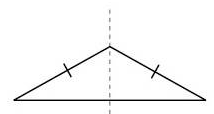This line of symmetry is also known as the altitude of an isosceles triangle.

(ii) A circle has an infinite number of lines of symmetry all along its diameters.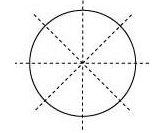#### Question 3:

Identify three examples of shapes with no line of symmetry.

A scalene triangle, a parallelogram and a trapezium do not have any line of symmetry.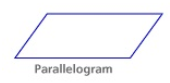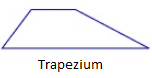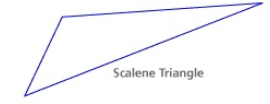#### Question 4:

Identify multiple lines of symmetry, if any, in each of the following figures: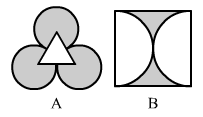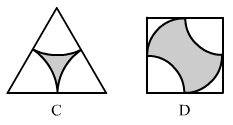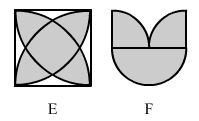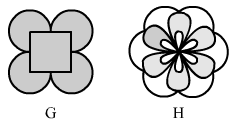(A) The given figure has 3 lines of symmetry. Therefore it has multiple lines of symmetry.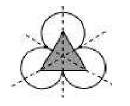(B) The given figure has 2 lines of symmetry. Therefore it has multiple lines of symmetry.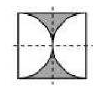(C) The given figure has 3 lines of symmetry. Therefore it has multiple lines of symmetry.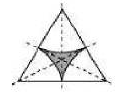(D) The given figure has 2 lines of symmetry. Therefore it has multiple lines of symmetry.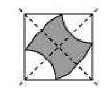(E) The given figure has 4 lines of symmetry. Therefore it has multiple lines of symmetry.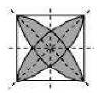(F) The given figure has only 1 line of symmetry.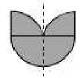(G) The given figure has 4 lines of symmetry. Therefore it has multiple lines of symmetry.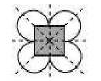(H) The given figure has 6 lines of symmetry. Therefore it has multiple lines of symmetry.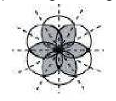97 docs

,

,

,

,

,

,

,

,

,

,

,

,

,

,

,

,

,

,

,

,

,

,

,

,

,

,

,

,

,

,

;